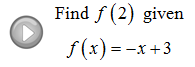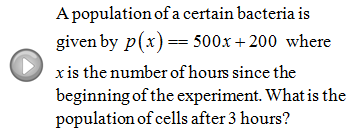## Pages

Showing posts with label cost. Show all posts
Showing posts with label cost. Show all posts

### Introduction to Functions

You may have noticed that we have been evaluating or "plugging-in" lots of numbers to find the corresponding y-values.  We have been doing this to find points on the graph (x, y).  This process can be streamlined using function notation.
This new notation above is read, "y is equal to f of x".  A function is a rule that uniquely assigns one output to every one input. So, at this point we can think of our non-vertical lines as functions.
The domain of a function is the set of inputs, usually the x-values.  The range of a function is the set of outputs, usually the y-values.
Evaluate the given function.
Find x.
Do not let the function notation discourage you, it takes some time to get used to.  The main thing to remember is that y = f(x); somtimes x is given and sometimes y or f(x) is given.

Graph the given linear function.
Word Problem. Bill has a popular software company which sells copies of its program for \$149.  If the initial start up cost for the company was \$10,000 and it costs \$12 to produce each copy:
a.  Find a cost function C(x) that models this business.
b.  Find a revenue function R(x) that models this business.
c.  Find the profit function P(x) using your functions above.
d.  Find the profit when 1000 programs are produced and sold.
e.  Find the number of programs that must be sold to break even.

Solution:
a. Cost Function - Include all fixed and variable costs of production.
b. Revenue Function - Include all proceeds from sales.
c. Profit Function - Revenue less cost of production.
d. Here x = 1,000 programs produced and sold.
e. Break Even - Occurs when profit is equal to zero.
Video Examples on YouTube:### Simplifying Algebraic Expressions

The properties of real numbers are very important in our study of Algebra. These properties can be applied in Algebra because a variable is simply a letter that represents a real number. The distributive property is one that we apply often when simplifying algebraic expressions. Given real numbers a, b, c:
a(b + c) = ab + ac
When multiplying an expression within parentheses you must multiply everything inside by the number or variable that you are distributing.

Simplify.
When simplifying, we will often have to combine like terms after we distribute.  This step is consistent with the order of operations, multiplication before addition.
---